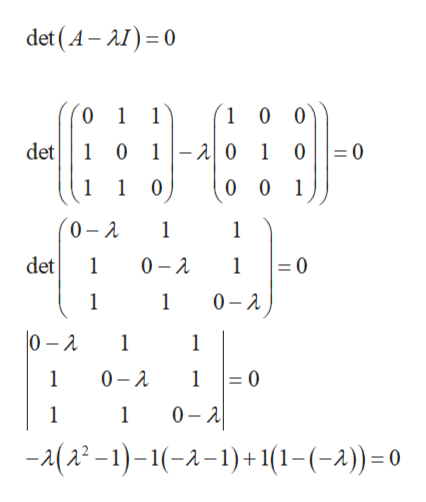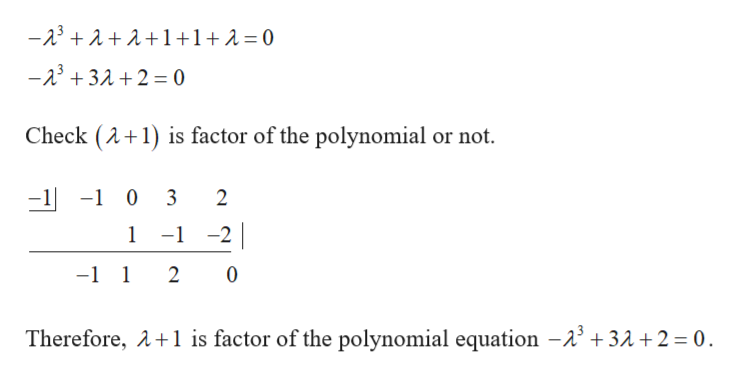Question
12 views

Find the general solution to given set of first order equations.

check_circle

Step 1

The given set of first order equation is,

Step 2

Find the Eigen values of the matrix.help_outlineImage Transcriptionclosedet (A-AI)0 0 1 1 10 0 1 0 12 0 1 0= 0 det 1 0 0 0 1 0 A 1 1 det 1 0 A 1 =0 1 0 A 1 0-2 1 1 1 0 A 1 0 0 A 1 -(2-)-1(-2-)+1(1-(-2))= 0 fullscreen
Step 3

On further simpli...help_outlineImage Transcriptionclose-23 1+1+ 2 = 0 -5322= 0 Check (A1) is factor of the polynomial or not. 0 3 2 1 --2 -1 1 2 0 Therefore, A1 is factor of the polynomial equation -A3 +32 + 2 = 0. fullscreen

### Want to see the full answer?

See Solution

#### Want to see this answer and more?

Solutions are written by subject experts who are available 24/7. Questions are typically answered within 1 hour.*

See Solution
*Response times may vary by subject and question.
Tagged in

### Math